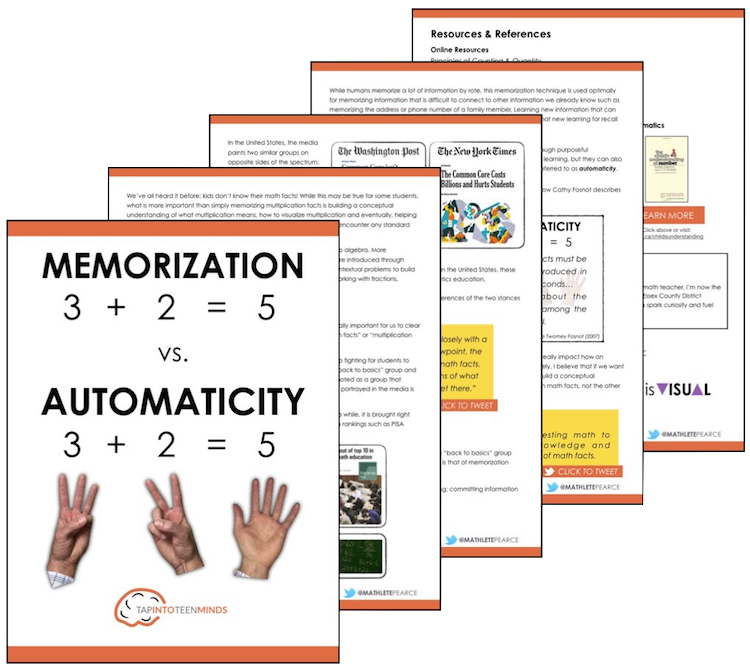MPM1D Unit 1 – Relations

Practice interpreting linear equations.  I.e.: if linear trends are increasing or decreasing, identify values of x given a value of y

MPM1D Unit 2 – Polynomials

Sec. 2.1 (3.2) – Work With Exponents – Practice 1

Practice exponents of 0 and 1.  Good refresher.

Sec. 2.1 (3.2) – Work With Exponents – Practice 2

Practice bases which are fractions.  Note some questions have negative exponents which we do not cover.  Click the “hint” button to learn something new.

Sec. 2.2 (3.3) – Discover the Exponent Laws

Practice using exponent laws when given powers with the same base

Sec. 2.3 (3.3) – More Exponent Laws

Practice simplifying expressions using exponent laws

Sec. 2.5 (3.5) – Collect Like Terms – Practice 1

Practice collecting like terms with basic polynomials.  Good refresher!

Sec. 2.7 (3.7) – The Distributive Property

Practice the basic concept of the Distributive Property

Sec. 2.7 (3.7) – Distributive Property & Simplification By Collecting Like Terms

Practice using distribution, then collect like terms to simplify the polynomial.

Sec. 2.8 (3.8) – Adding and Subtracting Polynomials

Practice simplifying by adding and subtracting polynomials

MPM1D Unit 3 – Equations

Sec. 3.1 (4.1) – Solve Simple Equations 1

Practice solving one-step linear equations

Sec. 3.1 (4.1) – Solve Simple Equations 2

Practice solving two-step linear equations

Sec. 3.2 (4.2) – Solve Multi-Step Equations

Practice solving more complex equations with more than two steps

Sec. 3.3 (4.3) – Solving Equations With Fractions

Practice solving equations that involve one or more fractions

Sec. 3.5 (4.5) – Modelling With Algebra – Writing Expressions

Practice taking math sentences and creating mathematical expressions to represent the scenario.

Practice taking math sentences and creating mathematical expressions to represent the scenario in more difficult situations. These are more difficult than what you would expect from this course, but practicing these can give you an added edge.

MPM1D Unit 4 – Modelling With Graphs

Sec. 4.3 (5.3) – Slope of a Line

Practice finding slope of a linear relation

Sec. 4.4 (5.4) – Slope as a Rate of Change

Practice finding the rate of change for real world scenarios

Sec. 4.7.R – Modelling With Graphs

Practice graphing linear relations given an equation

MPM1D Unit 5 – Analyse Linear Relations

Sec. 5.1 (6.1) – Slope Y-Intercept Form, y = mx + b

Practice finding equations in slope y-intercept form

Sec. 5.2 (6.2) – Standard Form, Ax + By + C = 0

Convert between slope y-intercept form and standard form

Sec. 5.3 (6.3) – Graph a Line Using Intercepts

Practice solving for the x-intercept of a linear relation

Sec. 5.5 (6.5) – Equations With Slope and a Point

Practice finding the y-intercept when given slope and a point

MPM1D Unit 6 – Geometric Relationships

Sec. 6.1 (7.1) – Angles in Triangles – Angle Types

Practice your skills at identifying acute, obtuse, and right angles

Sec. 6.1 (7.1) – Angles in Triangles – Triangle Types

Practice identifying whether triangles are equilateral, isosceles, or scalene

Sec. 6.1 (7.1) – Angles in Triangles – Find Angles

Practice determining the missing angle in each triangle

Sec. 6.1 (7.1) – Angles in Triangles – Complementary & Supplementary

Practice using your knowledge of complementary and supplementary angles

Sec. 6.3 (7.3) – Angle Relationships – F, Z, C Patterns

Practice using your knowledge of F, Z, & C Patterns

Sec. 6.3 (7.3) – Angle Relationships – Solve for Unknown

Practice connecting algebra to geometry.  Use geometric relationships to solve for an unknown variable.

Sec. 6.3 (7.3) – Angle Relationships – More Difficult 1

Practice more difficult problems which require a firm understanding of different geometric relationships

Sec. 6.3 (7.3) – Angle Relationships – More Difficult 2

Tougher still…

Sec. 6.3 (7.3) – Angle Relationships – Polygons

Practice sum of interior angles of a polygon knowledge 180(n-2)

MPM1D Unit 7 – Measurement Relationships

Sec. 7.3-7.5 – Volume and Surface Area of Prisms and Pyramids

Practice volume and surface area

Memorization vs. Automaticity

What's The Difference?I’m Kyle Pearce and I am a former high school math teacher. I’m now the K-12 Mathematics Consultant with the Greater Essex County District School Board, where I uncover creative ways to spark curiosity and fuel sense making in mathematics. Read more.

Not What You're Looking For?

Search By Criteria:

Spark Curiosity!